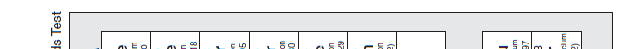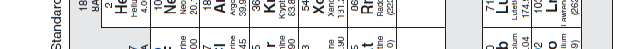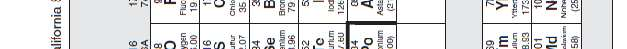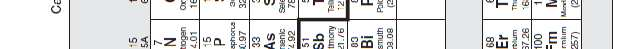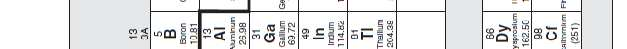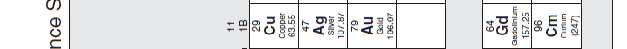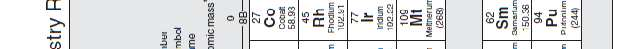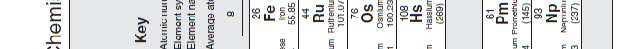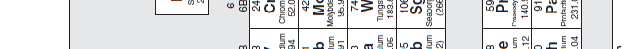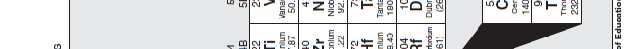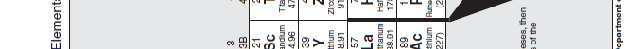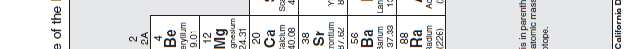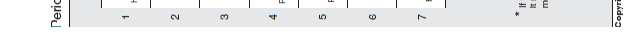## Entrance exam spring 2010 part b

PROBLEMS
12.4.2010
Entrance examination for degree programme in
maritime management

Instructions
The duration of the examination is 2 h 45 min
There are 10 statements to be marked false or true. The maximum score is 5 points. Part 2 (Mathematics + Logical deduction + physics/chemistry)
There are 10 problems to solve, each worth 3 points. For each problem, write the answer in the space provided on the answer sheet, C, and include calculation details whenever the solution requires calculation. Whenever solving the problems 8 through 10, you have to choose either the one in physics or the one in chemistry, if you solve both, the one with lower score will count. Do not turn the page until you are given the permission to do
so.

The problems are the following:
a) Simplify the expression [1 – 3(x + 2)] - 2x c) Evaluate the following expression for m = 3 and n = -1: 3݉ଶ െ a) Solve the following equation for x: x – 2(1 – x) = 2(1 + 3x) + 1 b) Solve the following equation for m: ܹ ൌ ݉ ݒଶ 3. A bookstore reduced the price of a book with 25%. After that the sale rose by 10%. With how many per cent rose the number of sold books? 4. In the trapezium below the angle A and B are 90° and the angle C 102°. AB is 9.1 m and BC is 3.2 m. Calculate a) DC and b) the area of the trapezium. 5. A person makes a cubical object of steel. Inside the object is a ball-shaped hollow room which is as big as possible and so that the steel´s least thickness is 5.5 mm. The length of the side of the cube is 210.0 mm. Calculate the weight of the object. Steel´s density is 7.87 kg/dm3. The volume of the ball-room can be calculated by the formula ܸ ൌ ߨݎଷ , r = radius of the ball. 6. Fill in the table with the positive integer numbers a, b, c and d using the tips 1 to 4. Tip 1: On row 1 there is only three different numbers. Tip 2: The sum of the numbers in column A is 2/3 of the sum of the numbers of Tip 3: The sum of the numbers in column D is 66. Tip 4: The sum of the numbers in row 3 is a multiple of 23. 7. A box, whose weight is 182 kg, is drawn with constant speed 21 m along a horizontal concrete floor. The kinetic friction between the box and the floor is 0.58. Calculate the work done when the box is drawn with a horizontal force. (acceleration due to gravity, g = 9,81 m/s2). When solving any of the problems 8 through 10, you have to choose either the one in physics (A) or the one in chemistry (B). If you solve both, the one with lower score will count. 8A. A lamp is marked 12V/10W. The lamp is connected to 24V accumulator. In series with lamp you must then connect a resistor. Calculate the resistance of the resistor. 8B. Caffeine, a stimulant found in coffee, tea, chocolate, and some medications, contains 49.48% carbon, 5.15% hydrogen, 28.87% nitrogen, and 16.49% oxygen by mass and has a molar mass of 194.2. Determine the molecular formula of caffeine. 9A. In an electric kettle with good heat insulation 0.85 kg water is warmed by an electrical resistance of 25 Ω, which is connected to a voltage of 230 V. In what time will the temperature of the water rise by 20 °C? The specific heat capacity of water is 4190 J/(kg°C) 9B. Baking soda (NaHCO3) is often used as an antacid. It neutralizes excess hydrochloric acid secreted by the stomach: NaHCO3(s) + HCl(aq) → NaCl(aq) + H2O(l) + CO2(aq) Milk of magnesia, which is an aqueous suspension of magnesium hydroxide, is also used as an antacid: Mg(OH)2(s) + 2HCl(aq) → 2H2O(l) + MgCl2(aq) Which is the more effective antacid per gram, NaHCO3 or Mg(OH)2? 10A. A meteorological balloon has volume of 20.0 m3 and a weight of 10.5 kg (gas included). What amount of cargo in kg can the balloon lift? The density of the air is 1.18 kg/m3 and acceleration of gravity 9.81 m/s2. 10B. Quicklime (CaO) is produced by the thermal decomposition of calcium carbonate (CaCO3). Calculate the volume of CO2 produced at STP from the decomposition of 152 g of CaCO3 according to the reaction